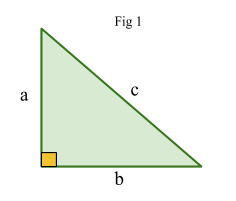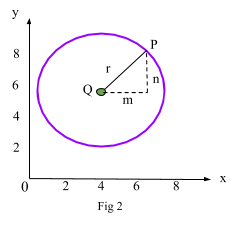# Equation Of A Circle

Contents

## Summary

Formula for equation of a circle is:${ (x\quad -\quad a) }^{ 2 }\quad +\quad { (y\quad -\quad b) }^{ 2 }\quad =\quad { r }^{ 2 }$

Where (a, b) is the center of the circle and r is the radius.

Before we move on to the equation of a circle, let’s just recap Pythagoras theorem from your GCSE studies:

We know that Pythagoras Theorem can be applied when we have a right angle triangle with a missing length of any side.

i.e refer to Fig 1 where:a is the length of the triangle,

b is the width of the triangle &

c is the hypotenuse

The formula for Pythagoras theorem is:$a^{ 2 }\quad +\quad { b }^{ 2 }\quad =\quad { c }^{ 2 }$

Pythagoras theorem plays a key role in deriving the equation of a circle.

#### The equation of a Circle

Look at the circle in Fig 2.The centre of the circle is Q (4, 6).

The radius of the circle is r which is the length from Q to P where point P is (x, y).

We can find the value of r using the pythagorean theorem as a right angle triangle is formed with height n and width m:$m^{ 2 }\quad +\quad { n }^{ 2 }\quad =\quad { r }^{ 2 }$

We can see that lengths$n\quad =\quad y\quad -\quad 6$  and$m\quad =\quad x\quad -\quad 4$.

Now substituting the values in the formula above we get:${ (y\quad -\quad 6) }^{ 2 }\quad +\quad { (x\quad -\quad 4) }^{ 2 }\quad =\quad { r }^{ 2 }\quad \quad \Rightarrow$   This is the equation of the circle in Fig 2

In general, a circle with radius r and center (a, b) has the equation:${ (x\quad -\quad a) }^{ 2 }\quad +\quad { (y\quad -\quad b) }^{ 2 }\quad =\quad { r }^{ 2 }$

#### Example #1

Q. What is the center and radius of the circle with equation:${ (x\quad -\quad 5) }^{ 2 }\quad +\quad { (y\quad -\quad 7) }^{ 2 }\quad =\quad { 25 }$

Solution:

From the equation we can see that the center of the circle is:

(a, b)  = (5, 7)

And the radius of the circle is:${ r }^{ 2 }\quad =\quad 25$$r\quad =\quad \sqrt { 25 }$$r\quad =\quad 5$

#### Example #2

Q. Write down the equation of the circle in Fig 3.Solution:

From the diagram, we can see that center of the circle is Q(8, 6) and the radius is r = 2

Putting the above values in the general formula for equation of a circle, we get:${ (x\quad -\quad 8) }^{ 2 }\quad +\quad { (y\quad -\quad 6) }^{ 2 }\quad =\quad { (2) }^{ 2 }$

Ans:${ (x\quad -\quad 8) }^{ 2 }\quad +\quad { (y\quad -\quad 6) }^{ 2 }\quad =\quad { 4 }$

##### Reference:

CGP AS-level mathematics edexcel revision and practice book